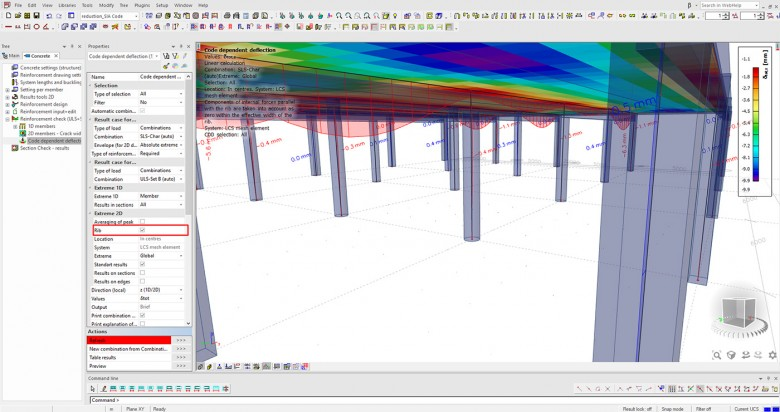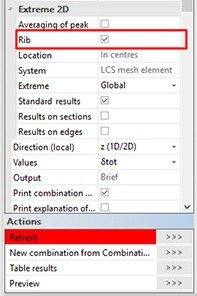# Determining long term deflections in slabs with ribs

Date:
Monday, 19 November, 2018
Region:
InternationalRibbed slabs are a common structural element in nowadays’ buildings. The system is widely used in multi-story buildings where the beam and the slab are cast at the same time. Specific requirements should be considered for the calculation of stiffness of such a system.

With SCIA Engineer 18.1 you can use a new procedure for considering the effect of the rib action in the calculation of the final stiffness of the cross-section that is generated based on the slab-beam connection. This new procedure can be deactivated in the settings giving you the possibility to compare the result for the rib action taken into account and the rib action neglected.

## A NEW METHODOLOGY FOR THE CALCULATION OF LONG-TERM DEFLECTION OF SLABS WITH RIBS

In version 18.1 you can define a beam as a rib in your floor/slab system and the software itself calculates the accurate stiffness for the rib and the slab independently. Moreover, you obtain both the total deflection and the deflection due to creep at the same time.
The calculation of long-term deflections takes into account the stiffness of the final cross-section (part of the slab + the beam) with the internal forces recalculated to the centre of gravity of that cross-section.

## Procedure of calculation

### First scenario: Ribs are not taken into account

If the rib action is not considered, then the deflection is calculated separately for the beam (a member with eccentricity) and for the slab. The following assumptions apply for the calculation of beam’s deflection:

• Only the cross-section (rib cross-section) defined in the “rib” properties is taken into account.
• The forces in the centre of gravity of the rib cross-section are used.
• Only longitudinal reinforcement inside the rib cross-section is considered.

For the calculation of the deflection of the slab, the following assumptions apply:

• The forces in the centre of each finite element are used.
• The reinforcement considered equals the sum of reinforcement defined in the slab (2D reinforcement meshes, free bars) and the reinforcement transformed from the flange of the final beam cross-section.

### SECOND SCENARIO: RIBS ARE TAKEN INTO ACCOUNT

If the rib action is considered, then the deflection is calculated for the equivalent cross-section.

The final cross-section of the rib is divided into two or three (for a rib aligned to the centre of the slab) entities. The equilibrium for the serviceability limit state is calculated for the whole final cross-section, but sectional characteristics and stiffness are calculated only for the selected entity.## CONCLUSION

There are several advantages stemming from the approach that takes the rib action into account (second scenario):

1. Transfer of the reinforcement inside the flange into standard reinforcement inside the slab for the calculation of the stiffness of the slab.
2. The new solution considers the following effects for the calculation of the stiffness of the equivalent cross-section:
o    The height of the compression zone is calculated for the quasi-permanent combination (value Xs).
o    The maximum tensile stress inside a slab entity of the final cross-section is calculated for the characteristic combination (crack forces, with tension).
o    The maximum tensile stress in the reinforcement in the whole cross-section is calculated for the quasi-permanent combination (stiffness forces, without tension).
o    The distribution coefficient for the whole cross-section is calculated for the quasi-permanent combination.
3. The stiffness of 2D reinforcement meshes inside the effective width is taken into account for the configuration with more than one rib.

To see the advantages in action, let’s consider a simple example of a single rib. The picture below compares two calculations. On the left, a part of a slab is modelled by a simple beam of T-shaped cross-section (with a one-meter wide flange) and analysed as pure 1D member. On the right, the model, analysed using the second scenario presented above, consists of one-meter wide stretch of a plate and a beam attached as a rib to the plate. It is clearly seen that both models produce very close results.

Visit SCIA Engineer help to read all the details and theoretical background for the calculation of code dependent deflections.